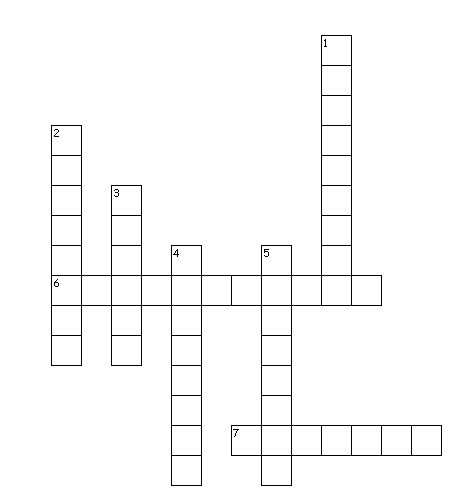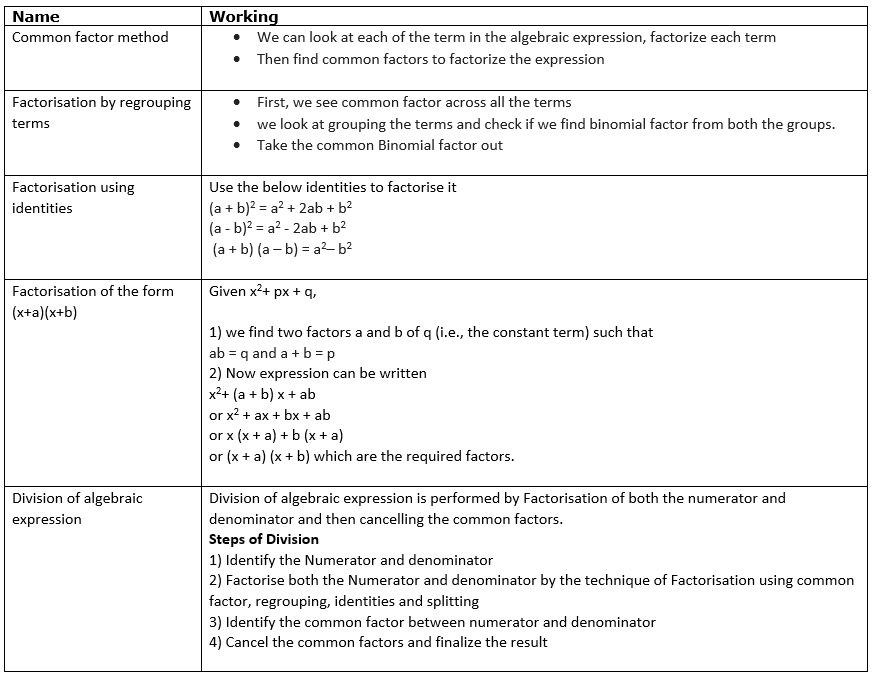# Factorisation Class 8 Notes

In this page we will explain the topics for the chapter 8 of Factorisation Class 8 Maths.We have given quality Factorisation Class 8 Notes along with video to explain various things so that students can benefits from it and learn maths in a fun and easy manner, Hope you like them and do not forget to like , social share and comment at the end of the page.

Table of Content

## Factors of Natural Number

Factors of Natural number are the number which can divide the Natural Number
Consider 16
We can write
$16= 2 \times 8= 4 \times 4=2 \times 2 \times 2 \times 2=1 \times 16$
So 1,2,4,8,16 all are factors of Natural Number 16. Prime Factors are the factors which are prime number.
A number can be expressed as product of prime factors and that form is called prime form
$16=2 \times 2 \times 2 \times 2$

## Factors of algebraic expression

An algebraic expression can also be expressed as Products of factors
Example
$6xy$
$6xy= 6 \times (xy) = 6 \times(x) \times (y)= 2 \times 3 \times (xy)= 2 \times 3 \times(x) \times (y)$
We can see that 2,3,x,y cannot be further expressed as products of factor.  So they are called the prime factors. We called them irreducible factors in terms of algebraic expression

## Factorisation of algebraic expression

When we factorise an algebraic expression, we write it as a product of factors. These factors may be numbers, algebraic variables or algebraic expressions
The expression 6x (x - 2). It can be written as a product of factors. 2,3, x and (x - 2)
6x (x - 2).  =2×3× x× (x - 2)
The factors 2,3, x and (x +2) are irreducible factors of 6x (x + 2).

## Method of Factorisation

### Common factor method

1) We can look at each of the term in the algebraic expression, factorize each term into irreducible factors
2) Then find common factors to factorize the expression

Example
1) $2x+4$
Here we can see 2 is the common factor in the terms
=$2(x+2)$
2) $11x^2y +5x$
First reduce it to irreducible factors form
$=11 \times(x) \times (x) \times y+5 \times (x)$
Now we can see that x is the common factor
$=x(11xy+5)$

### Factorisation by regrouping terms

1) First we see common factor across all the terms in the algebraic expression
2) we look at grouping the terms and check if we find binomial factor from both the groups.
3) Take the common Binomial factor out
Example
1) $2xy + 3x + 2y + 3$
First reduce it to irreducible factors form. There is no common factor across all the terms
$= 2 \times x \times y + 3 \times x + 2 \times y + 3$
Now we think about grouping the terms. We can see that we have common factor between first two terms
$= x \times (2y + 3) + 1 \times (2y + 3)$
$= (2y + 3) (x + 1)$

2) $6xy - 4y + 6 - 9x$
There are no common factors across all the terms, so we think of grouping and rearranging the terms
$=6xy - 4y - 9x+6$
$=2y(3x-2) -3(3x-2)$
$=(2y-3)(3x-2)$

### Factorisation using identities

We can use the below identities to factorise algebraic expression
$(a + b)^2 = a^2 + 2ab + b^2$
$(a - b)^2 = a^2 - 2ab + b^2$
$(a + b) (a - b) = a^2 - b^2$

Example
1) $4x^2 - 12x + 9$
We can write this as
$(2x)^2 -2 \times 2x \times 3 + 3^2$
Which resembles the second identity
Here a=2x and b=3
So
$4x^2 - 12x + 9 =(2x-3)^2$
2) $16x^2 -81$
We can write this as
(4x)2 -92
Which resembles the third identity
Here a=4x and b=9
So
$16x^2 -81=(4x-9)(4x+9)$

Practice Questions
• $z^{2} - 64$
• $y^{2} - 49$
• $x^{2} + 6 x + 9$
• $x^{2} + 14 x + 49$
• $x^{2} - 4 x + 4$
• $x^{2} + 2 x + 1$

Watch this tutorial for more example on Factorisation

### Factorisation of the form $(x+a)(x+b)$

Given x2+ px + q,
1) we find two factors a and b of q (i.e., the constant term) such that
ab = q and a + b = p
2) Now expression can be written
$x^2+ (a + b) x + ab$
or $x^2 + ax + bx + ab$
or $x(x + a) + b(x + a)$
or $(x + a) (x + b)$ which are the required factors.
Example
1)$x^2 - 7x+ 12$
Comparing to $x^2+ px + q$
We have p=-7 and q=12
Now $12 =(- 3) \times (-4)$ and (-3) + (-4) =- 7
$=x^2 - 3x - 4x + 12$
$= x (x - 3) - 4 (x - 3) = (x - 3) (x - 4)$

2))$y^2+ 11y+ 30$
Comparing to x2+ px + q
We have p=11 and q=30
Now 30 =( 5) × (6) and (5) + (6) =11
$=y^2+ 5y+6y+ 30$
$= y (y +5) +6 (y +5) = (y +5) (y + 6)$

Practice Questions
• $x^{2} - 18 x + 80$
• $y^{2} - 14 y + 40$
• $x^{2} + 4 x - 12$
• $z^{2} + z - 6$
• $x^{2} - 2 x - 3$
• $y^{2} + y - 2$
• $m^{2} - 36$
• $n^{2} + 2 n - 24$
• $x^{2} - 16 x + 63$
• $a^{2} - 4 a - 5$

## Division of algebraic expression

Division of algebraic expression is performed by Factorisation of both the numerator and denominator and then cancelling the common factors.
General Steps of Division
1) Identify the Numerator and denominator
2) Factorise both the Numerator and denominator by the technique of Factorisation using common factor, regrouping, identities and splitting
3) Identify the common factor between numerator and denominator
4) Cancel the common factors and finalize the result

Example
$\frac {48 (x^2yz + xy^2z + xyz^2)}{4xyz}$
$=\frac {48xyz (x + y + z)}{4xyz}$
$= \frac {4 \times 12 \times xyz (x + y + z)}{4xyz}$
$= 12 (x + y + z)$

Here Dividend=48 (x2yz + xy2z + xyz2)
Divisor=4xyz
Quotient=12 (x + y + z)

So, we have
Dividend = Divisor × Quotient.
In general, however, the relation is
Dividend = Divisor × Quotient + Remainder
When reminder is not zero

## Solved Example on Division

1)  34a2bc2  /  51 abc
Solution
=17×2×a×a×b×c×c / 17×3×a×b×c
Cancelling common factors
= 2ac/3

2) 36(x2– 7x+ 12)/2(x-4)
Solution
Here we need to factorize both the numerator and denominator
Let’s take a look at Numerator first
36(x2– 7x+ 12)
Here we can factorize this using splitting method
=36(x2 – 3x – 4x + 12)
=36(x-3)(x-4)
So division will become
36(x2– 7x+ 12)/2(x-4)
=36(x-3)(x-4)/2(x-4)
=18(x-3)

Watch this tutorial for more example on Division

## Common Mistake done in algebraic expression and equation

Mistake 1
Coefficient 1 of a term is usually not shown. So student often ignores that. But while adding like terms, we should include it in the sum.
Wrong Approach
$x+2x+3x$
$=5x$
We ignored the coefficient 1
Right Approach
$x+2x+3x$
$= (1) x+2x+3x$
$=6x$

Mistake 2
When you multiply the expression enclosed in a bracket by a constant (or a variable) outside, you usually applied the multiplication to first term only. This is wrong calculation. Each term of the expression has to be multiplied by the constant (or the variable).
Wrong Approach
$2(2x+3)$
$=4x+3$
We ignored the second term
Right Approach
$2(2x+3)$
$=4x+6$

Mistake 3
when you square a monomial, you often ignored the   numerical coefficient. This is wrong approach. The   numerical coefficient and each factor has to be squared.
Wrong Approach
$(2x)^2$
$=2x^2$
We ignored the numerical coefficient
Right Approach
$(2x)^2$
$=4x^2$

Mistake 4
when you square a binomial, you often do not apply the proper formula. This is wrong approach. The right formula should be used
Wrong Approach
$(2x+3)^2=4x^2 +9$
We did not use the proper formula
Right Approach
$(2x+3)^2$
$=4x^2+9+12x$

## Test your Knowlegde with Crossword PuzzleAcross
6. In algebraic expressions this is is used in place of prime
7. Terms can be written as product of its
Down
1. A polynomial with three terms
2. A polynomial with one terms
3. it is called the highest power of a polynomial
4. it is an equality, which is true for all values of the variables in the equality
5. A polynomial with two terms.

## Summary

Here is Factorisation Class 8 Notes Summary